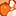# QlikView App Dev

Discussion Board for collaboration related to QlikView App Development.

Announcements
Support Cases coming to Qlik Community Oct. 4! Start chats, open cases, explore resources: READ DETAILS
cancel
Showing results for
Did you mean:Contributor

## Rank client on the basis of calendar input

Respected Grus.

I am using following expression to show top view of different channels (client)

=sum({\$<CHANNEL_NAME={"rank(aggr(sum(AMOUNT),CHANNEL_NAME))<=\$(V_ctop)"}>}AMOUNT)

In above expression V_ctop is input value coming from user (i.e. 2,3,4,5....).

Now I want user to also select TO and FROM date from calendar and storing in variables V_frmdte and V_todte respectively.

Say user want to see the top 5 channel w.r.t AMOUNT where invoice_date lies between 01-Jan-2017 to 31-Jul-2017 (stored in variables)

plz advice me how to include dates variables in above expression.

1 Solution

Accepted SolutionsMVP

Is this working for you?

=Sum({\$<CHANNEL_NAME={"rank(aggr(sum(AMOUNT),CHANNEL_NAME))<=\$(V_ctop)"}>}AMOUNT)

I would think not because you are missing an equal sign

=Sum({\$<CHANNEL_NAME={"=rank(aggr(sum(AMOUNT),CHANNEL_NAME))<=\$(V_ctop)"}>}AMOUNT)

=Sum({\$<CHANNEL_NAME={"=Rank(aggr(sum({<Date = {'>=\$(=V_frmdte)<=\$(=V_todte)'}>}AMOUNT),CHANNEL_NAME))<=\$(V_ctop)"}, Date = {'>=\$(=V_frmdte)<=\$(=V_todte)'}>}AMOUNT)

3 RepliesMVP

Is this working for you?

=Sum({\$<CHANNEL_NAME={"rank(aggr(sum(AMOUNT),CHANNEL_NAME))<=\$(V_ctop)"}>}AMOUNT)

I would think not because you are missing an equal sign

=Sum({\$<CHANNEL_NAME={"=rank(aggr(sum(AMOUNT),CHANNEL_NAME))<=\$(V_ctop)"}>}AMOUNT)

=Sum({\$<CHANNEL_NAME={"=Rank(aggr(sum({<Date = {'>=\$(=V_frmdte)<=\$(=V_todte)'}>}AMOUNT),CHANNEL_NAME))<=\$(V_ctop)"}, Date = {'>=\$(=V_frmdte)<=\$(=V_todte)'}>}AMOUNT)Contributor
Author

hi Sunny!!

Ya you were right '=' was typing mistake its actually there in my doc.

and so many thanks for your reply this is exactly I want...

cheers!!!MVP

You can close the thread Qlik Community Tip: Marking Replies as Correct or Helpful

Before develop something, think If placed (The Right information | To the right people | At the Right time | In the Right place | With the Right context)Community Browser